# Chemical kinetics Questions and Answers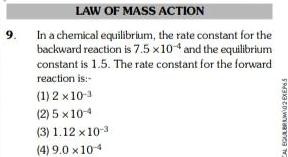Physical Chemistry
Chemical kinetics
9 LAW OF MASS ACTION In a chemical equilibrium the rate constant for the backward reaction is 7 5 x 104 and the equilibrium constant is 1 5 The rate constant for the forward reaction is 1 2x10 2 5 x 10 4 3 1 12 x 10 3 4 9 0 x 104 CAL EQUILIBRUM02EXEP65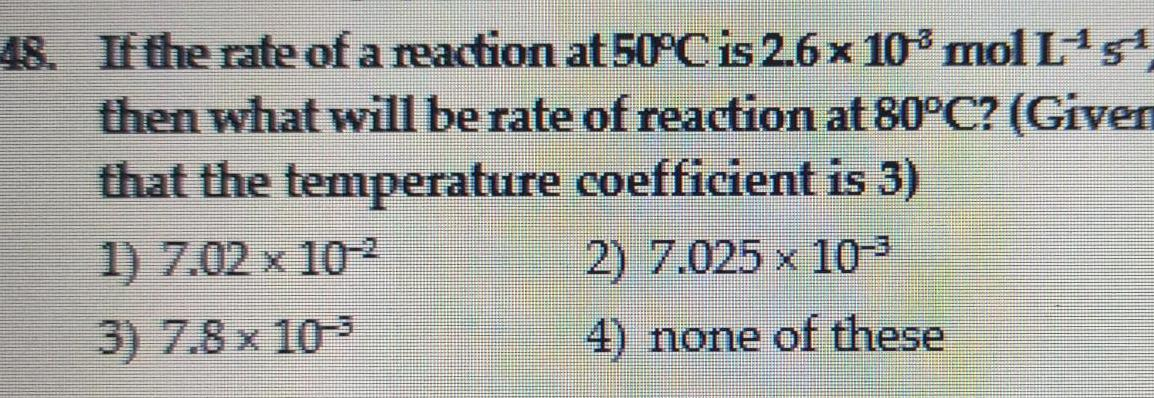Physical Chemistry
Chemical kinetics
48 If the rate of a reaction at 50 C is 2 6 x 10 mol L 5 then what will be rate of reaction at 80 C Given that the temperature coefficient is 3 1 7 02 x 10 2 7 025 x 10 3 7 8 x 10 4 none of these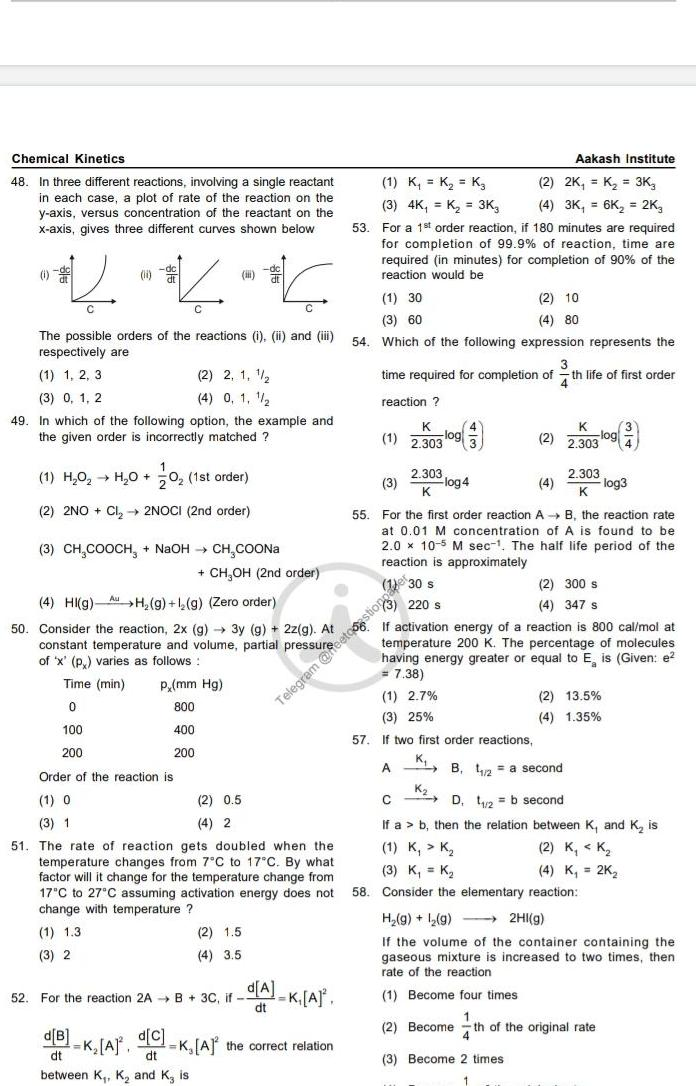Physical Chemistry
Chemical kinetics
Chemical Kinetics 48 In three different reactions involving a single reactant in each case a plot of rate of the reaction on the y axis versus concentration of the reactant on the x axis gives three different curves shown below 0 0 The possible orders of the reactions i ii and iii respectively are 1 1 2 3 2 2 1 1 4 0 1 1 2 3 0 1 2 49 In which of the following option the example and the given order is incorrectly matched 1 H O H O 0 1st order 2 2NO Cl 2NOCI 2nd order i 3 CH COOCH NaOH CH COONa K A CH OH 2nd order p mm Hg 800 400 200 d B d C dt dt between K K and K is 2 0 5 4 2 2 1 5 4 3 5 52 For the reaction 2A B 3C if Telegram K A K A the correct relation 4 HI g H g 1 g Zero order 50 Consider the reaction 2x g 3y g 2z g At constant temperature and volume partial pressurece 220 s of x p varies as follows Time min 0 100 200 Order of the reaction is 1 O 3 1 51 The rate of reaction gets doubled when the temperature changes from 7 C to 17 C By what factor will it change for the temperature change from 17 C to 27 C assuming activation energy does not change with temperature 1 1 3 3 2 d A dt 53 Aakash Institute 1 K K K 2 2K K 3K 3 4K K 3K3 4 3K 6K 2K3 For a 1st order reaction if 180 minutes are required for completion of 99 9 of reaction time are required in minutes for completion of 90 of the reaction would be 1 30 2 10 4 80 3 60 54 Which of the following expression represents the time required for completion of th life of first order 4 reaction K 1 2 303 2 303 K 3 log4 55 For the first order reaction A K 2 2 303 1 2 7 3 25 57 If two first order reactions A B the reaction rate at 0 01 M concentration of A is found to be 2 0 x 10 5 M sec The half life period of the reaction is approximately 1 30 s log 2 303 K log3 If activation energy of a reaction is 800 cal mol at temperature 200 K The percentage of molecules having energy greater or equal to E is Given e 7 38 2 300 s 4 347 s KB 2 a second K 2 13 5 4 1 35 C D 2 b second If a b then the relation between K and K is 1 K K 2 K K 3 K K 4 K 2K 58 Consider the elementary reaction H g 2 g 2HI g If the volume of the container containing the gaseous mixture is increased to two times then rate of the reaction 1 Become four times 2 Become th of the original rate 3 Become 2 times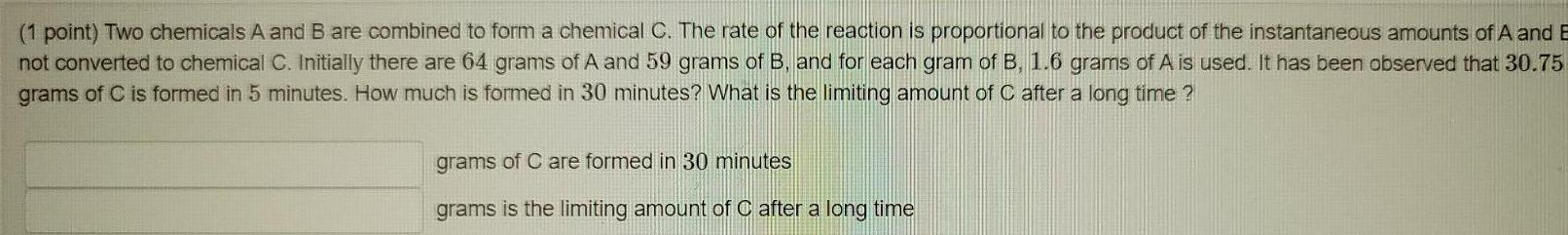Physical Chemistry
Chemical kinetics
1 point Two chemicals A and B are combined to form a chemical C The rate of the reaction is proportional to the product of the instantaneous amounts of A and B not converted to chemical C Initially there are 64 grams of A and 59 grams of B and for each gram of B 1 6 grams of A is used It has been observed that 30 75 grams of C is formed in 5 minutes How much is formed in 30 minutes What is the limiting amount of C after a long time grams of C are formed in 30 minutes grams is the limiting amount of C after a long time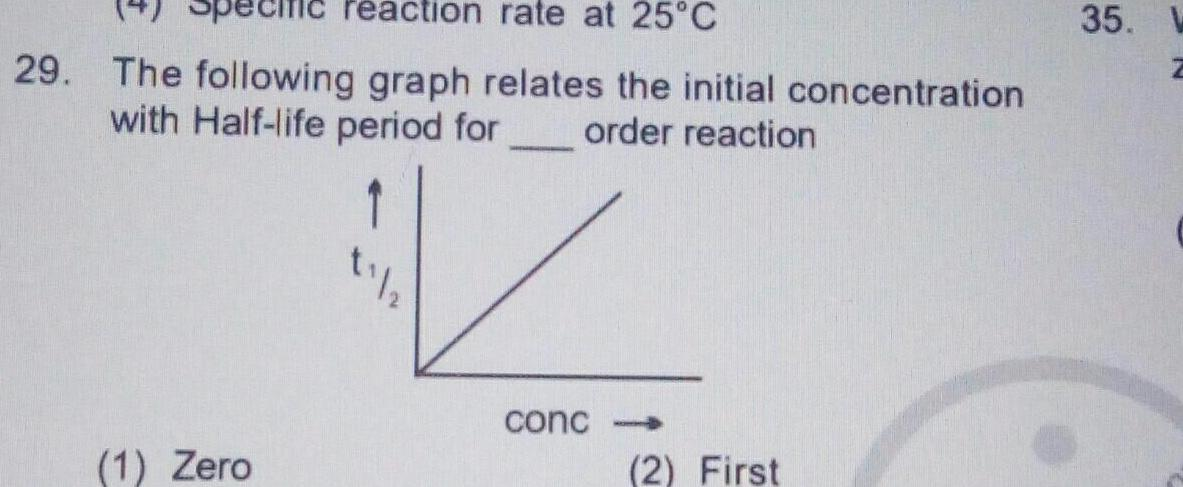Physical Chemistry
Chemical kinetics
reaction rate at 25 C 29 The following graph relates the initial concentration with Half life period for order reaction 1 Zero S 1 2 V conc 2 First 35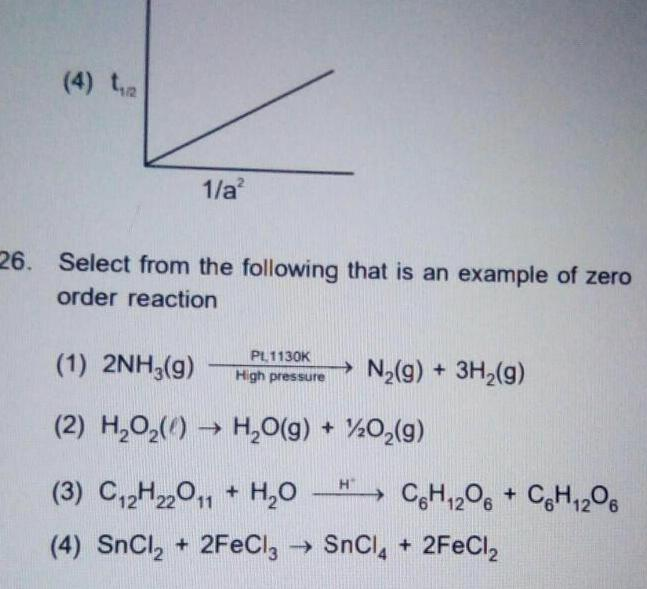Physical Chemistry
Chemical kinetics
4 2 1 a 26 Select from the following that is an example of zero order reaction 1 2NH3 g PL1130K High pressure 2 H O H O g O g 3 C 2H22O11 H O 4 SnCl 2FeCl3 SnCl 2FeCl N g 3H g H C6H12O6 C6H12O6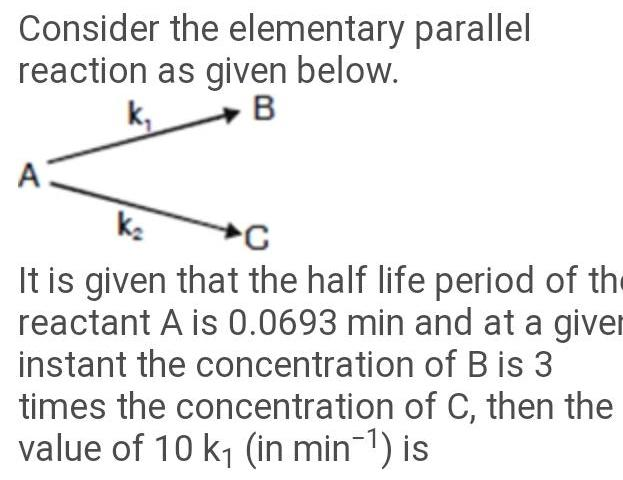Physical Chemistry
Chemical kinetics
Consider the elementary parallel reaction as given below B k A K C It is given that the half life period of the reactant A is 0 0693 min and at a giver instant the concentration of B is 3 times the concentration of C then the value of 10 k in min is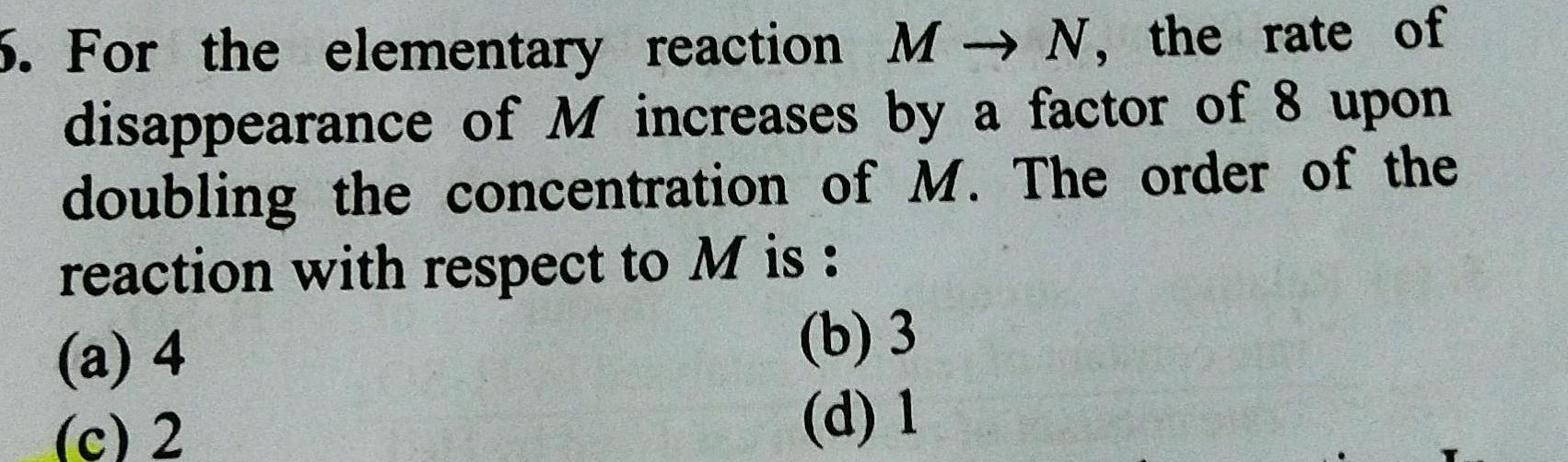Physical Chemistry
Chemical kinetics
5 For the elementary reaction MN the rate of disappearance of M increases by a factor of 8 upon doubling the concentration of M The order of the reaction with respect to M is b 3 d 1 a 4 c 2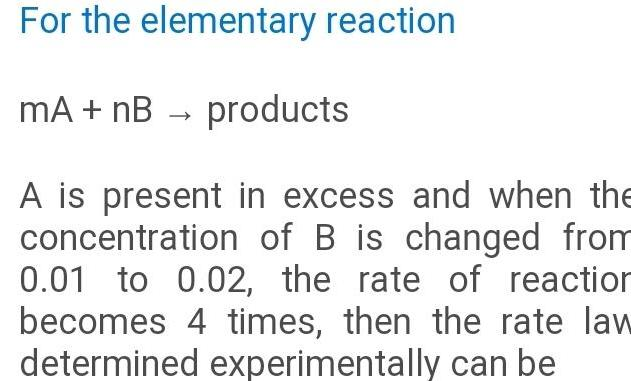Physical Chemistry
Chemical kinetics
For the elementary reaction mA nB products A is present in excess and when the concentration of B is changed from 0 01 to 0 02 the rate of reaction becomes 4 times then the rate law determined experimentally can be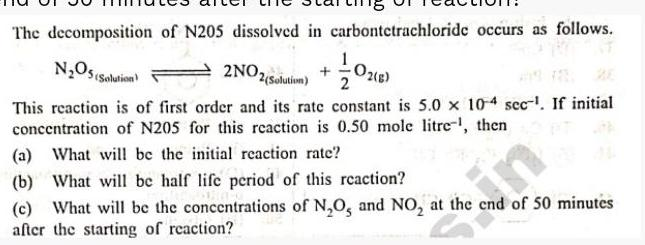Physical Chemistry
Chemical kinetics
The decomposition of N205 dissolved in carbontetrachloride occurs as follows N O5 Solution 2NO2 2 Solution O2 g 2 This reaction is of first order and its rate constant is 5 0 x 10 4 sec If initial concentration of N205 for this reaction is 0 50 mole litre then a What will be the initial reaction rate b What will be half life period of this reaction c What will be the concentrations of N O and NO at the end of 50 minutes after the starting of reaction in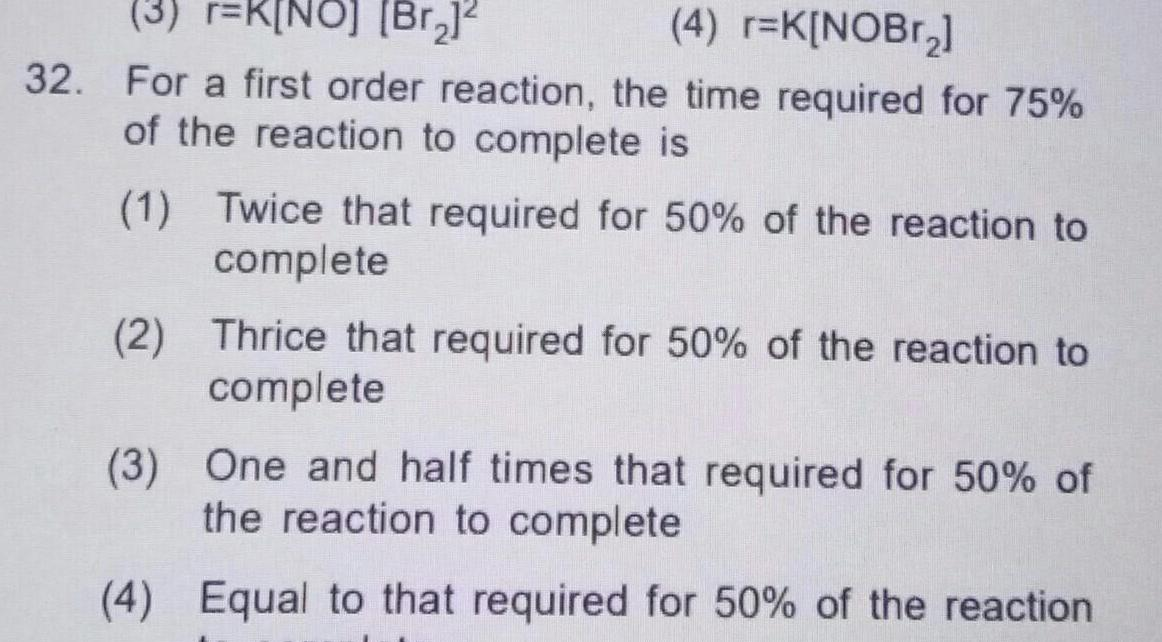Physical Chemistry
Chemical kinetics
3 r K NO Br 4 r K NOBr 32 For a first order reaction the time required for 75 of the reaction to complete is 1 Twice that required for 50 of the reaction to complete 2 Thrice that required for 50 of the reaction to complete 3 One and half times that required for 50 of the reaction to complete 4 Equal to that required for 50 of the reaction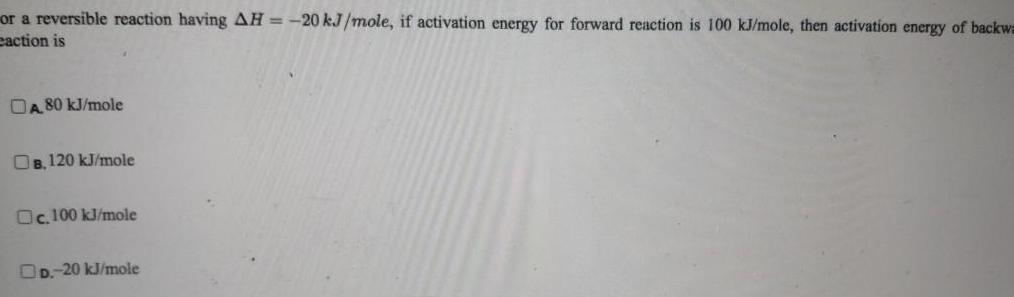Physical Chemistry
Chemical kinetics
or a reversible reaction having AH 20 kJ mole if activation energy for forward reaction is 100 kJ mole then activation energy of backwa eaction is A 80 kJ mole OB 120 kJ mole Oc 100 kJ mole DD 20 kJ mole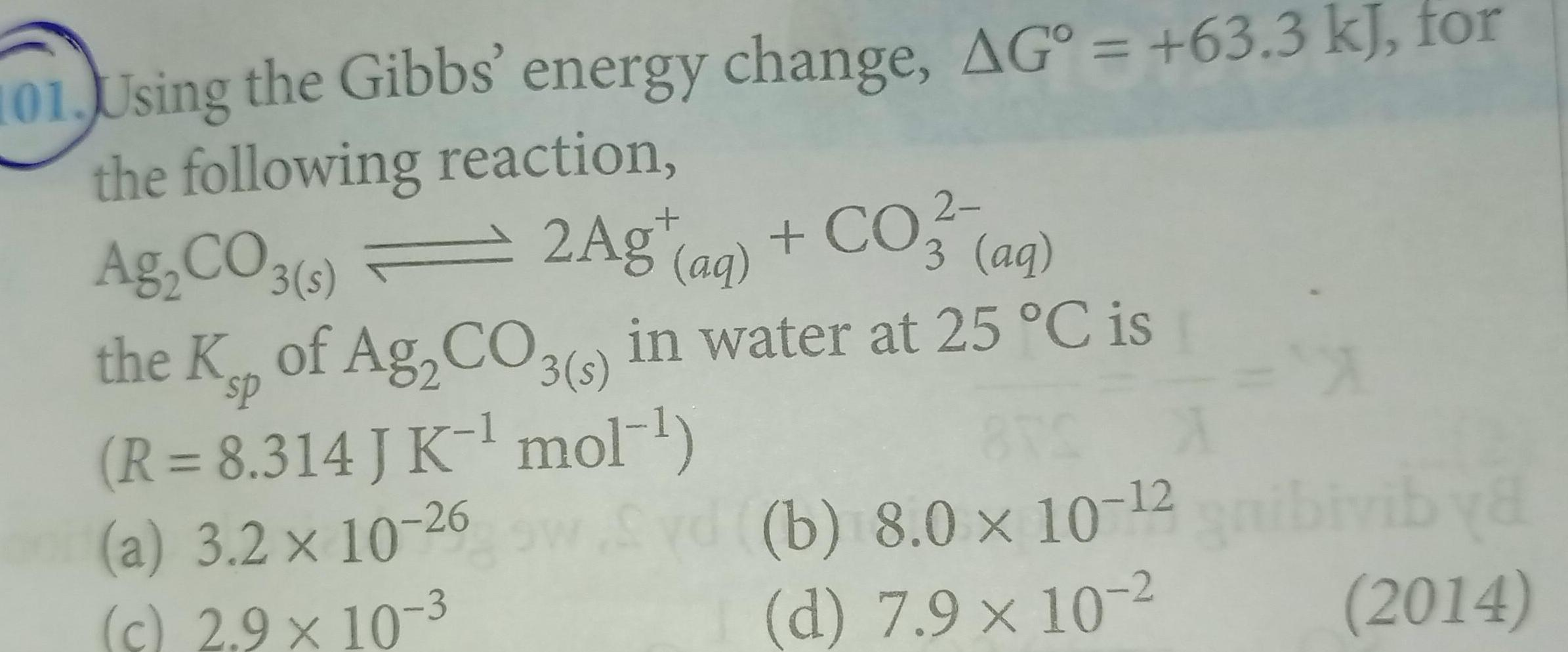Physical Chemistry
Chemical kinetics
101 Using the Gibbs energy change AG 63 3 kJ for the following reaction 2 Ag CO3 s 2Ag aq CO3 3 aq the Ksp of Ag CO3 s in water at 25 C is R 8 314 J K mol a 3 2 x 10 26 c 2 9 10 3 Syd b 8 0 x 10 2 gnibivib ya d 7 9 x 10 2 2014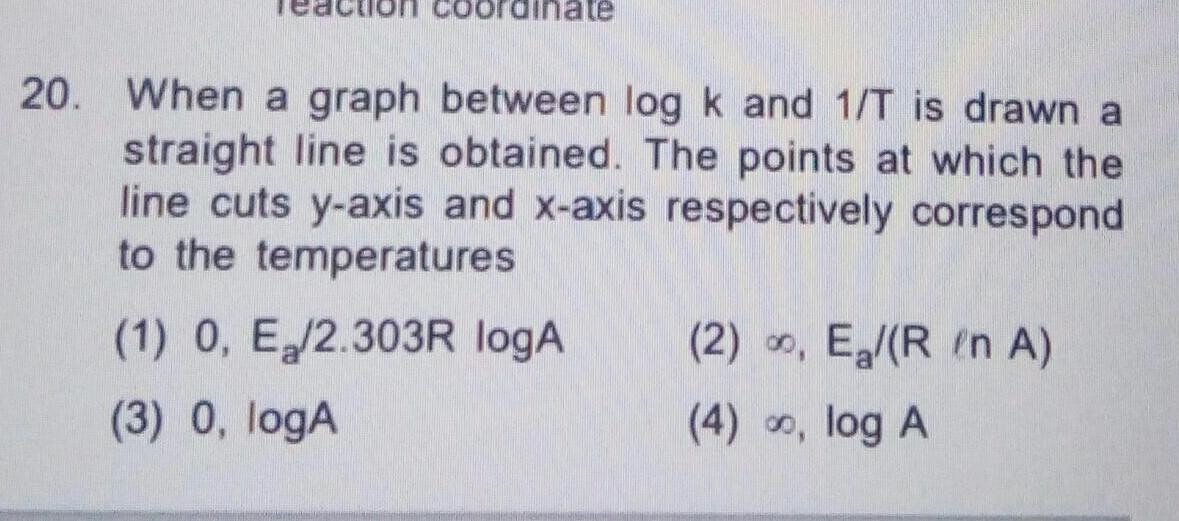Physical Chemistry
Chemical kinetics
Inate 20 When a graph between log k and 1 T is drawn a straight line is obtained The points at which the line cuts y axis and x axis respectively correspond to the temperatures 1 0 E 2 303R logA 3 0 logA 2 E R in A 4 log A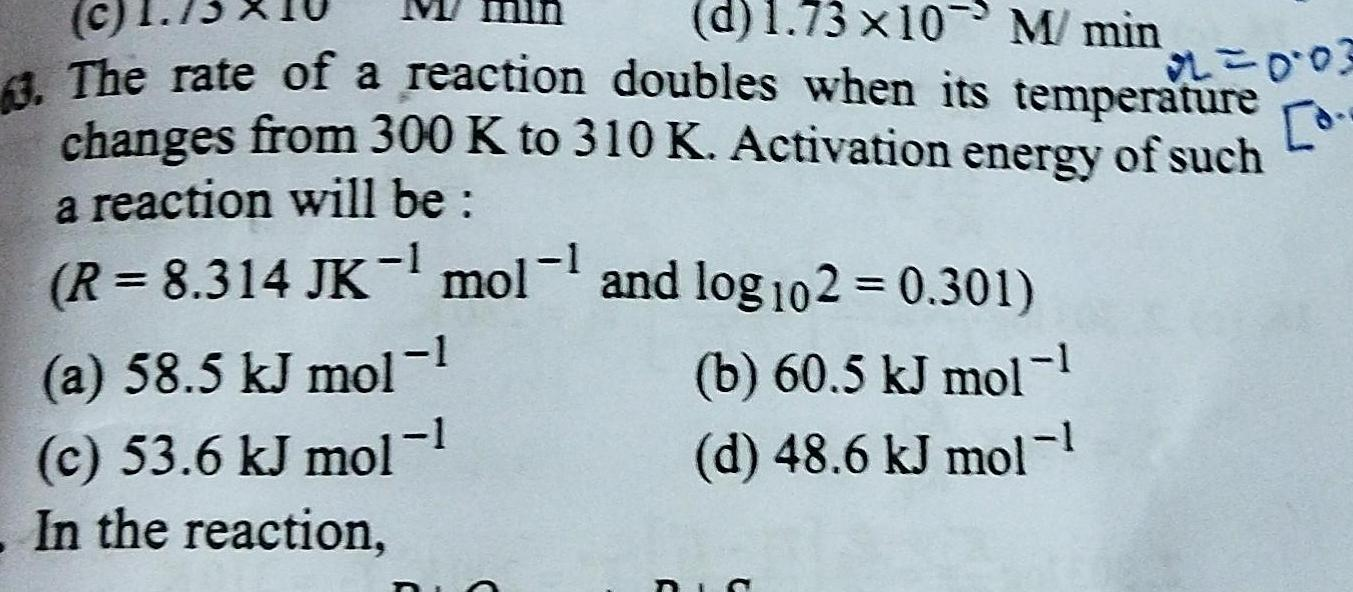Physical Chemistry
Chemical kinetics
c d 1 73x10 M min 3 The rate of a reaction doubles when its temperature changes from 300 K to 310 K Activation energy of such a reaction will be R 8 314 JK mol and log102 0 301 1 a 58 5 kJ mol 1 c 53 6 kJ mol 1 In the reaction b 60 5 kJ mol 1 d 48 6 kJ mol 1 DIS 22 0 03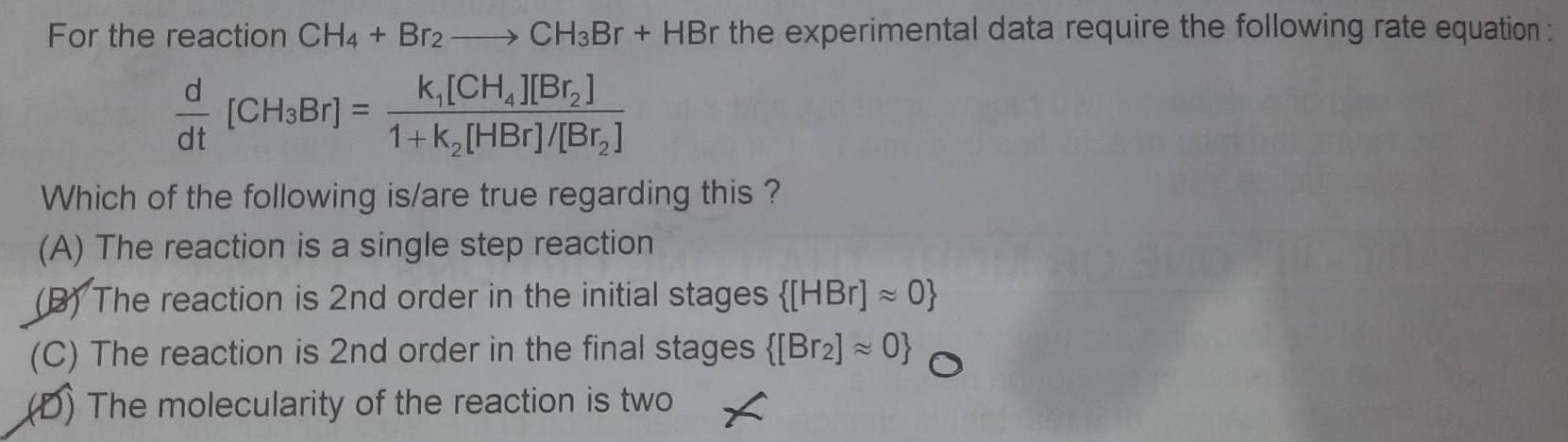Physical Chemistry
Chemical kinetics
For the reaction CH4 Br2CH3Br HBr the experimental data require the following rate equation k CH Br HBr Br d dt CH3Br 1 k Which of the following is are true regarding this A The reaction is a single step reaction BY The reaction is 2nd order in the initial stages HBr 0 C The reaction is 2nd order in the final stages Br2 0 The molecularity of the reaction is two f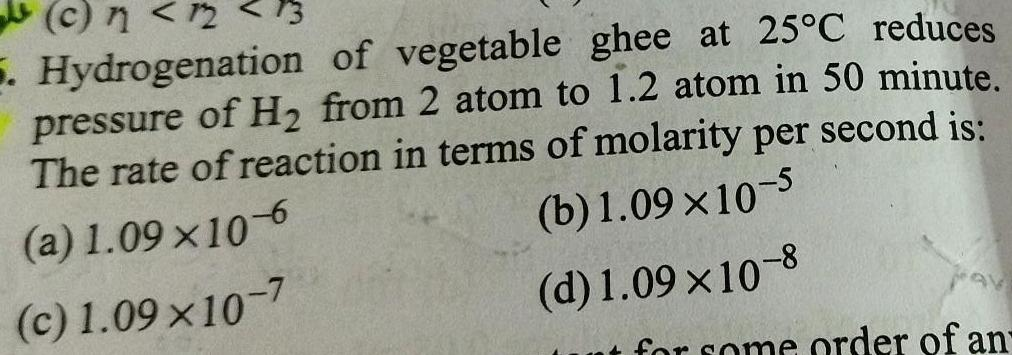Physical Chemistry
Chemical kinetics
13 c 12 5 Hydrogenation of vegetable ghee at 25 C reduces pressure of H from 2 atom to 1 2 atom in 50 minute The rate of reaction in terms of molarity per second is a 1 09 x10 6 b 1 09 10 5 c 1 09 10 7 d 1 09 10 8 ut for some order of an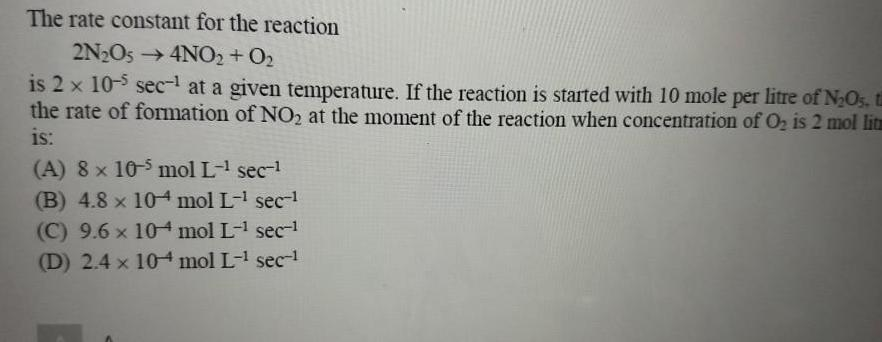Physical Chemistry
Chemical kinetics
The rate constant for the reaction 2N O5 4NO2 0 is 2 x 10 5 sec at a given temperature If the reaction is started with 10 mole per litre of N O5 t the rate of formation of NO at the moment of the reaction when concentration of O is 2 mol litm is A 8 x 105 mol L sec B 4 8 x 104 mol L sec C 9 6 x 104 mol L sec 1 D 2 4 x 104 mol L sec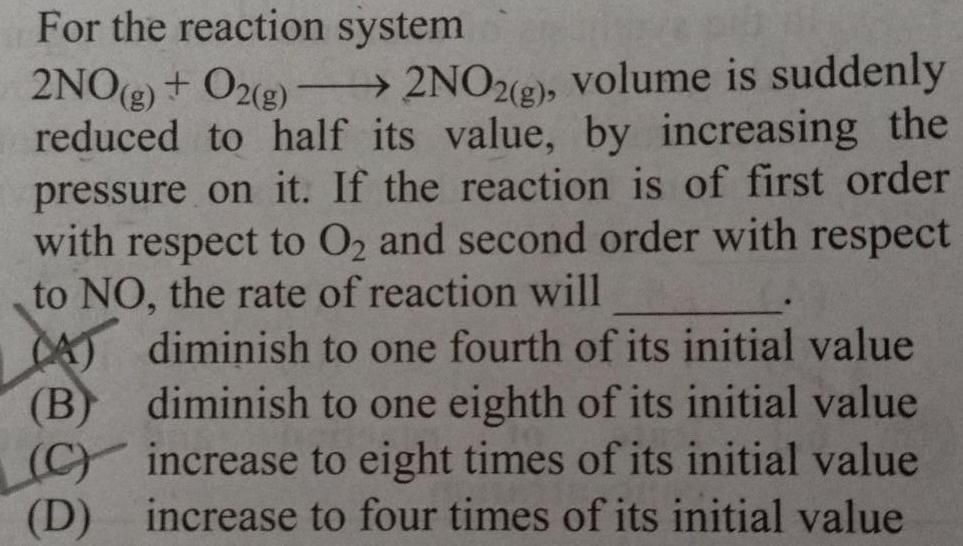Physical Chemistry
Chemical kinetics
For the reaction system 2NO g O2 g 2NO2 g volume is suddenly reduced to half its value by increasing the pressure on it If the reaction is of first order with respect to O and second order with respect to NO the rate of reaction will A B diminish to one fourth of its initial value diminish to one eighth of its initial value C increase to eight times of its initial value D increase to four times of its initial value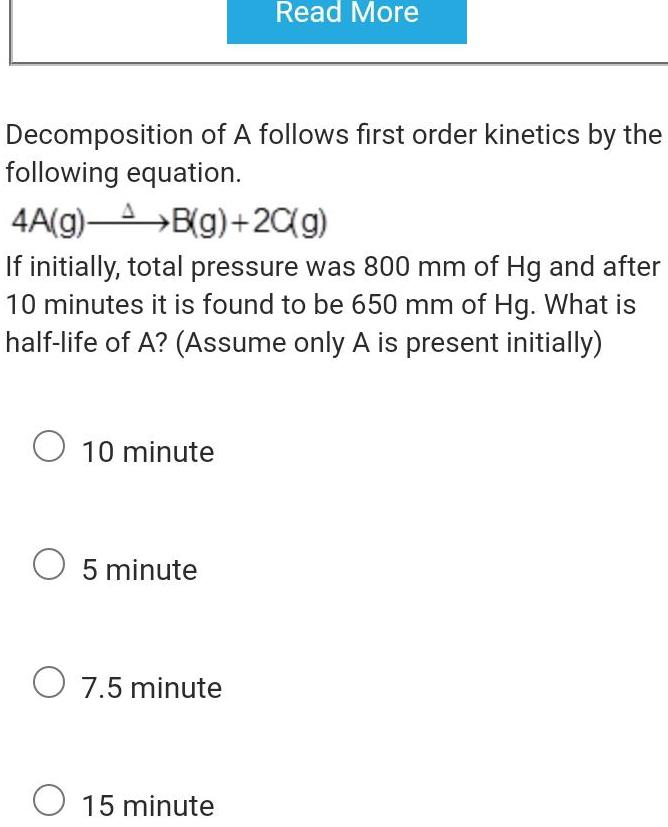Physical Chemistry
Chemical kinetics
Decomposition of A follows first order kinetics by the following equation 4A g B g 2C g If initially total pressure was 800 mm of Hg and after 10 minutes it is found to be 650 mm of Hg What is half life of A Assume only A is present initially O 10 minute O 5 minute Read More O 7 5 minute O 15 minute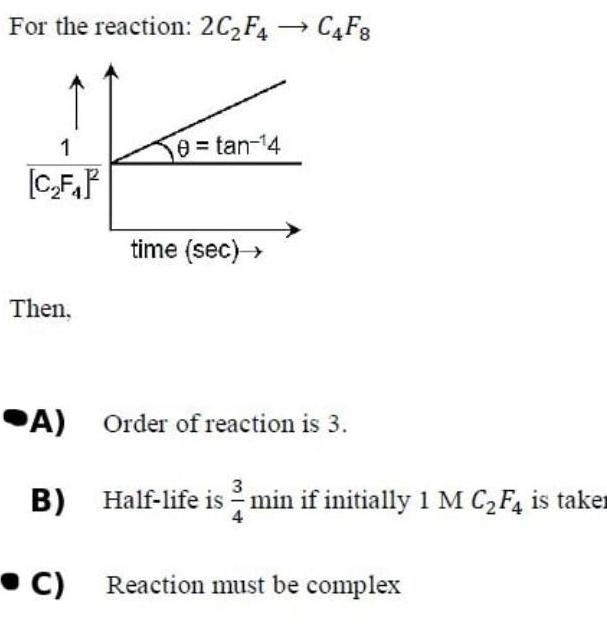Physical Chemistry
Chemical kinetics
For the reaction 2C F4C4F8 FANTA e tan 4 1 C F Then A B C time sec Order of reaction is 3 Half life is min if initially 1 M C F4 is take Reaction must be complex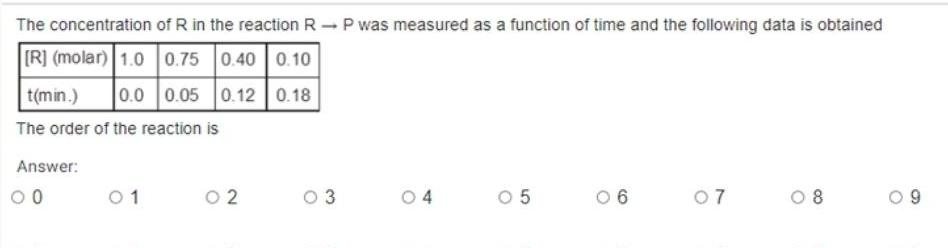Physical Chemistry
Chemical kinetics
The concentration of R in the reaction RP was measured as a function of time and the following data is obtained R molar 1 0 0 75 0 40 0 10 t min 0 0 0 05 0 12 0 18 The order of the reaction is Answer 00 01 02 0 3 04 05 6 07 08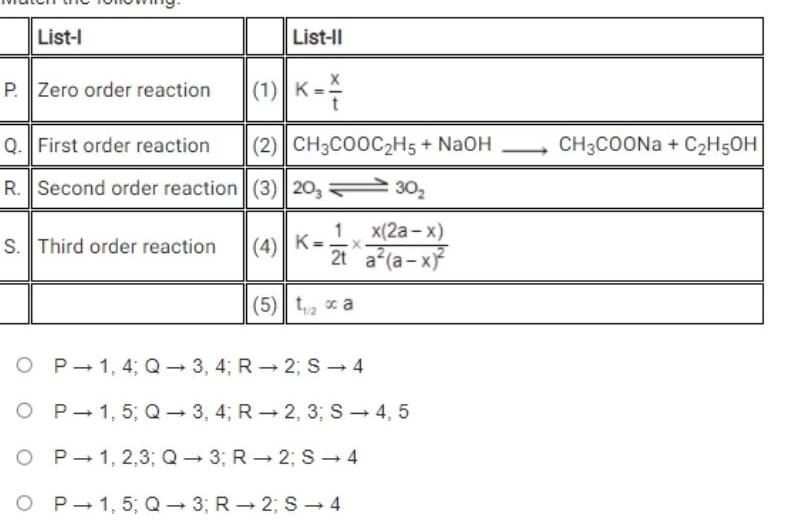Physical Chemistry
Chemical kinetics
List I P Zero order reaction Q First order reaction R Second order reaction S Third order reaction List II 1 K 2 CH3COOC H5 NaOH 3 20 30 x 2a x 2t a a x 4 K X 5 ta OP 1 4 Q3 4 R2 S 4 O P 1 5 Q3 4 R2 3 S 4 5 OP1 2 3 Q3 R2 S 4 O P 1 5 Q3 R 2 S 4 CH3COONa C H5OH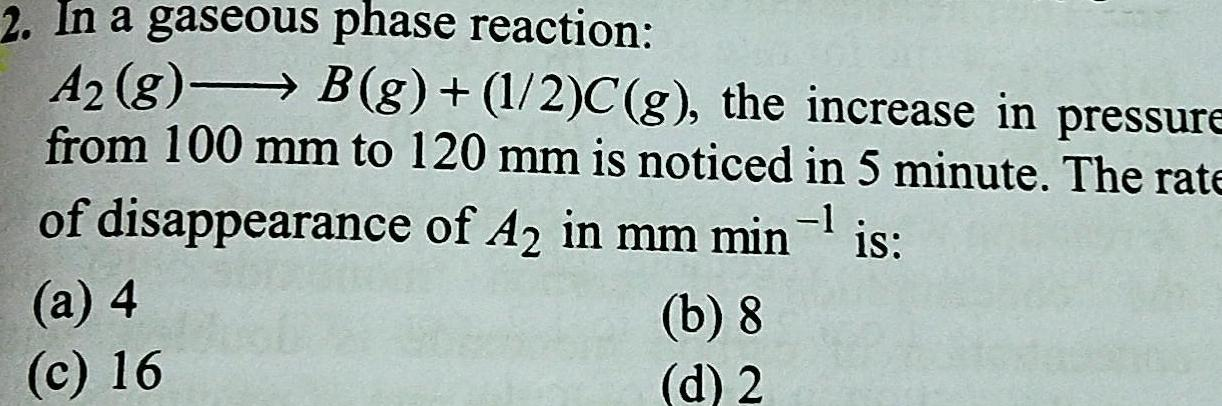Physical Chemistry
Chemical kinetics
2 In a gaseous phase reaction A g B g 1 2 C g the increase in pressure from 100 mm to 120 mm is noticed in 5 minute The rate of disappearance of A2 in mm min is a 4 c 16 b 8 d 2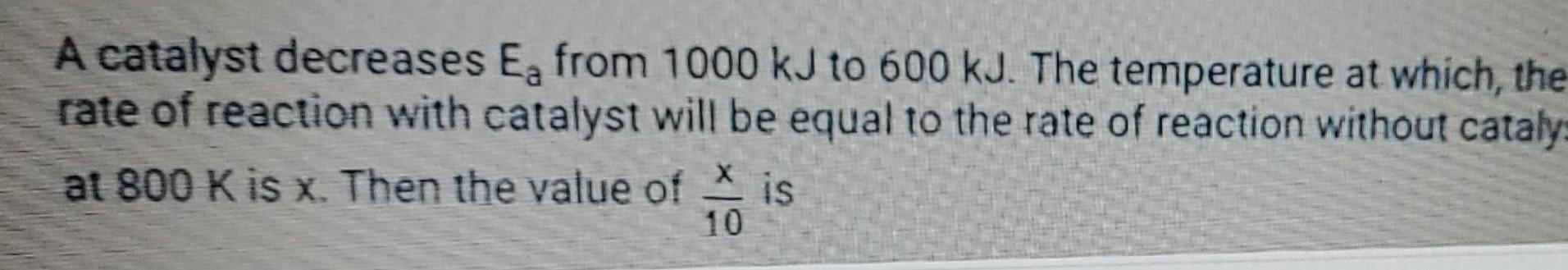Physical Chemistry
Chemical kinetics
A catalyst decreases Ea from 1000 kJ to 600 kJ The temperature at which the rate of reaction with catalyst will be equal to the rate of reaction without catalys at 800 K is x Then the value of is X 10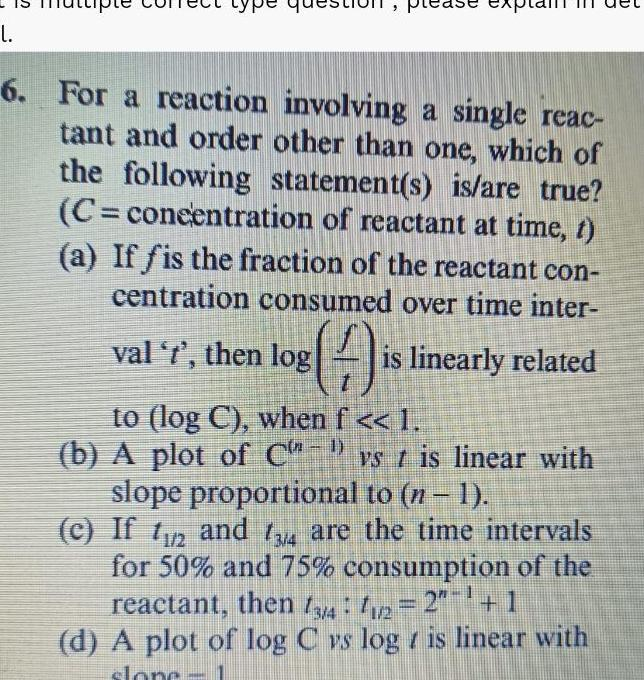Physical Chemistry
Chemical kinetics
L 6 For a reaction involving a single reac tant and order other than one which of the following statement s is are true C concentration of reactant at time t a If fis the fraction of the reactant con centration consumed over time inter val t then log A is linearly related to log C when f 1 b A plot of C vs 1 is linear with slope proportional to n 1 c If 112 and 134 are the time intervals for 50 and 75 consumption of the reactant then 13 4 1 2 2 1 d A plot of log C vs log is linear with slone 1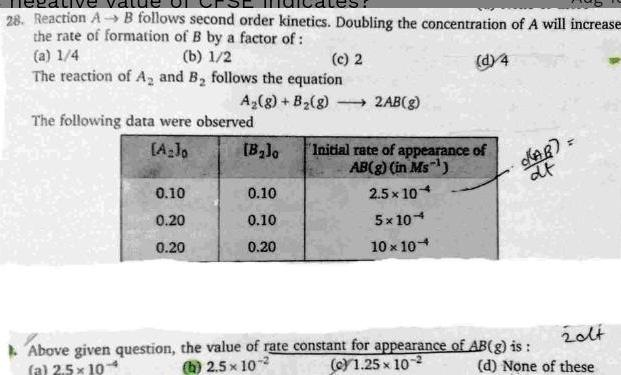Physical Chemistry
Chemical kinetics
28 Reaction A B follows second order kinetics Doubling the concentration of A will increase the rate of formation of B by a factor of a 1 4 b 1 2 c 2 d 4 The reaction of A and B follows the equation The following data were observed A Jo 0 10 0 20 0 20 A g B g 2AB g Above given question a 25 10 B 0 10 0 10 0 20 Initial rate of appearance of AB g in Ms 2 5 x 10 5x104 10 x 104 AB at 20lt d None of these the value of rate constant for appearance of AB g is b 2 5x 10 c 1 25x102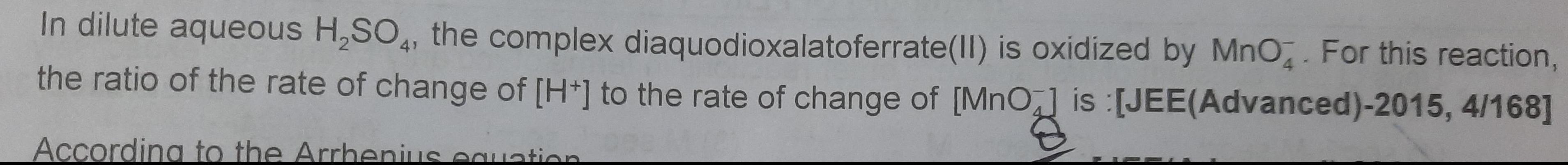Physical Chemistry
Chemical kinetics
In dilute aqueous H SO4 the complex diaquodioxalatoferrate II is oxidized by MnO4 For this reaction the ratio of the rate of change of H to the rate of change of MnO is JEE Advanced 2015 4 168 According to the Arrhenius equation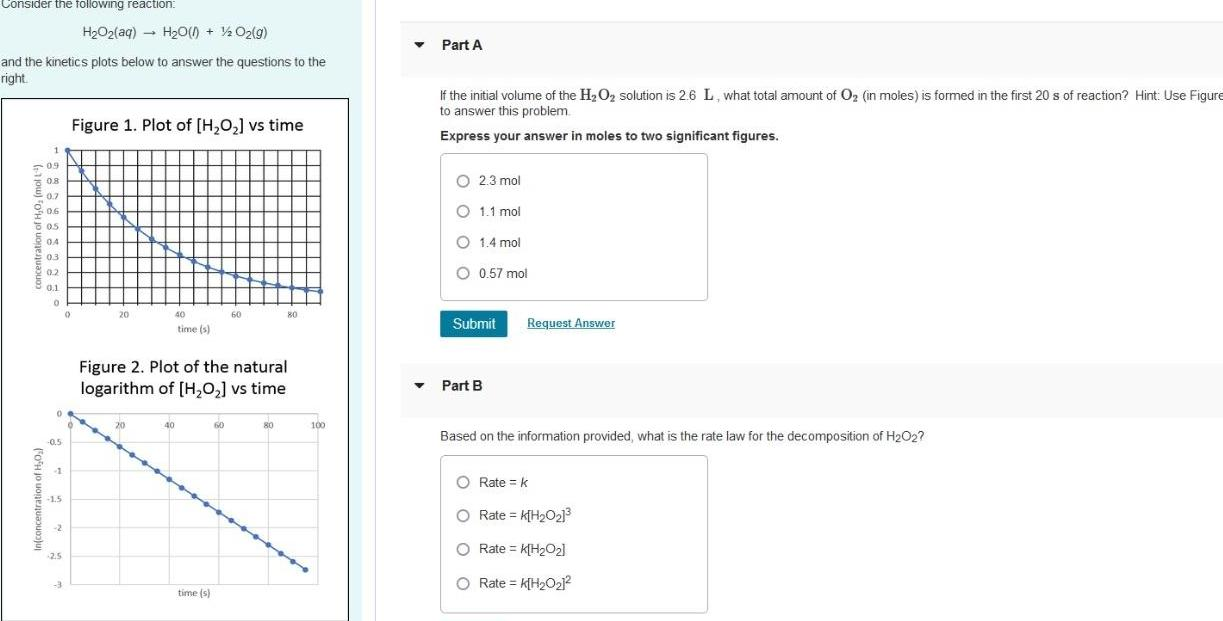Physical Chemistry
Chemical kinetics
Consider the following reaction and the kinetics plots below to answer the questions to the right 1 09 0 8 0 7 0 6 8 0 5 8 0 4 O 0 03 0 2 0 1 0 In concentration of H O 0 5 1 1 5 2 2 5 3 H O2 aq H O l 1 2O g O Figure 1 Plot of H O vs time 20 40 time s 40 Figure 2 Plot of the natural logarithm of H O vs time time s 60 60 80 80 100 Part A If the initial volume of the H O solution is 2 6 L what total amount of O in moles is formed in the first 20 s of reaction Hint Use Figure to answer this problem Express your answer in moles to two significant figures O 2 3 mol 1 1 mol O 1 4 mol O 0 57 mol Submit Part B Request Answer Based on the information provided what is the rate law for the decomposition of H O2 O Rate k O Rate K H O O Rate K H 0 O Rate K H 0 1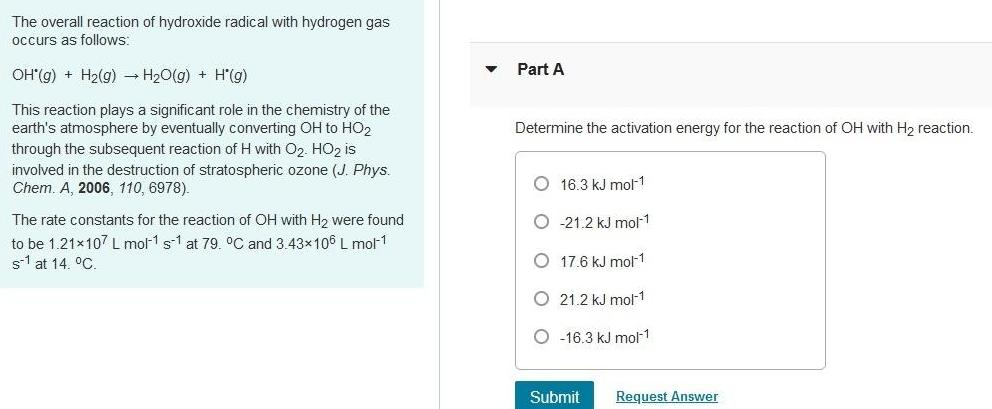Physical Chemistry
Chemical kinetics
The overall reaction of hydroxide radical with hydrogen gas occurs as follows OH g H g H O g H g This reaction plays a significant role in the chemistry of the earth s atmosphere by eventually converting OH to HO2 through the subsequent reaction of H with O HO2 is involved in the destruction of stratospheric ozone J Phys Chem A 2006 110 6978 The rate constants for the reaction of OH with H were found to be 1 21x107 L mol 1 s1 at 79 C and 3 43 106 L mol 1 s1 at 14 C Part A Determine the activation energy for the reaction of OH with H2 reaction O 16 3 kJ mol 1 O 21 2 kJ mol 1 O 17 6 kJ mol 1 O21 2 kJ mol 1 16 3 kJ mol 1 Submit Request Answer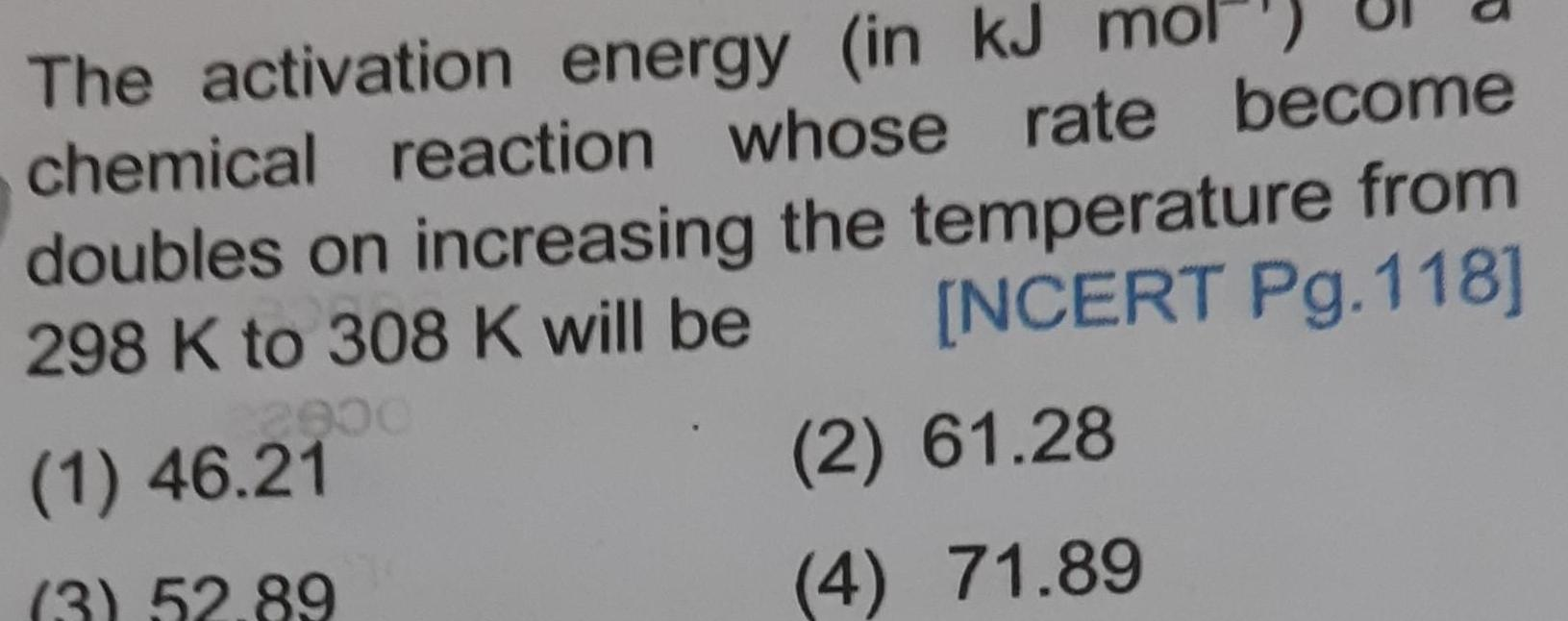Physical Chemistry
Chemical kinetics
The activation energy in kJ m chemical reaction whose rate become doubles on increasing the temperature from 298 K to 308 K will be NCERT Pg 118 1 46 21 3 52 89 2 61 28 4 71 89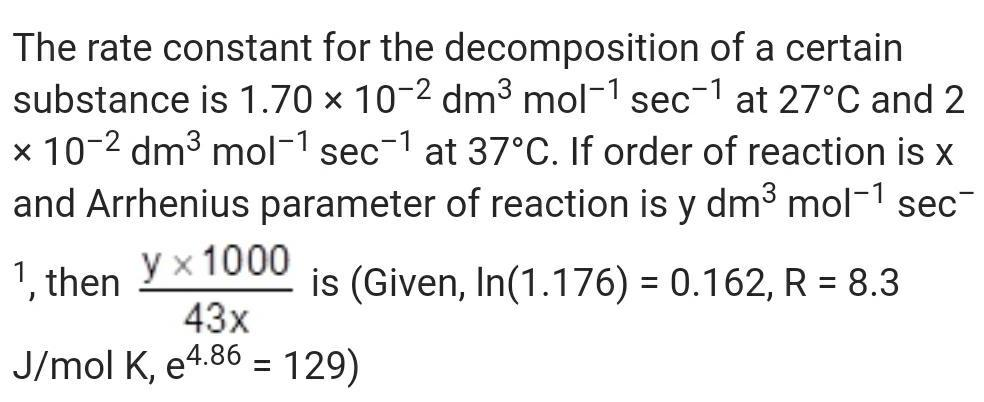Physical Chemistry
Chemical kinetics
The rate constant for the decomposition of a certain substance is 1 70 x 10 2 dm mol 1 sec1 at 27 C and 2 x 10 2 dm mol 1 sec1 at 37 C If order of reaction is x and Arrhenius parameter of reaction is y dm mol sec 1 then y 1000 is Given In 1 176 0 162 R 8 3 43x J mol K e4 86 129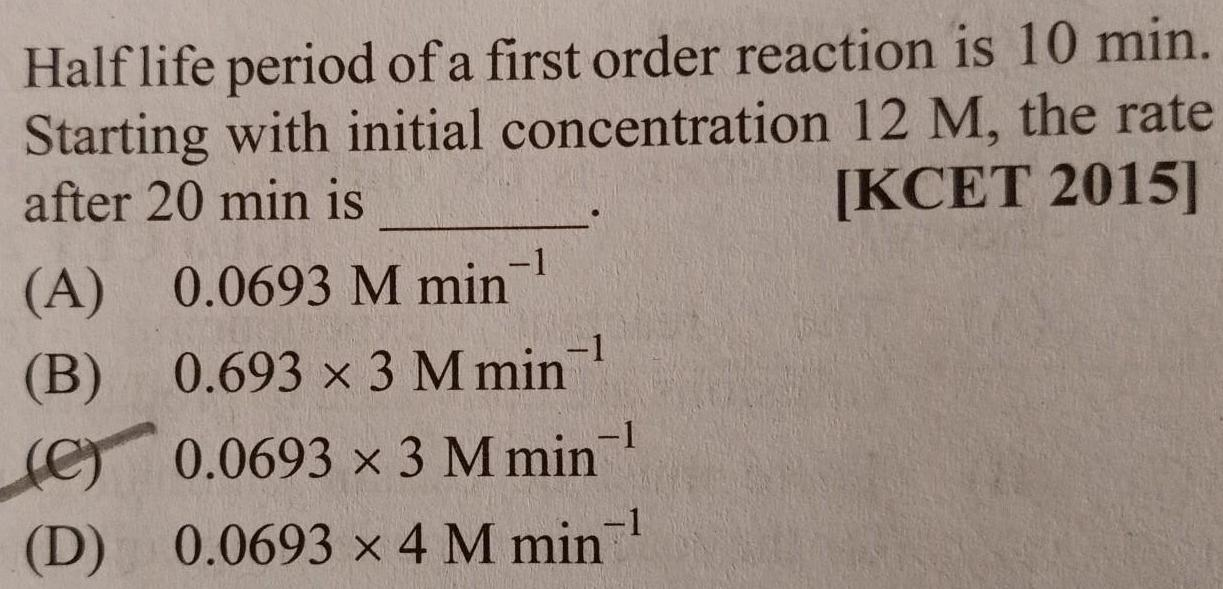Physical Chemistry
Chemical kinetics
Half life period of a first order reaction is 10 min Starting with initial concentration 12 M the rate after 20 min is KCET 2015 A 0 0693 M min B 0 693 x 3 M min 1 C 0 0693 x 3 M min D 0 0693 x 4 M min 1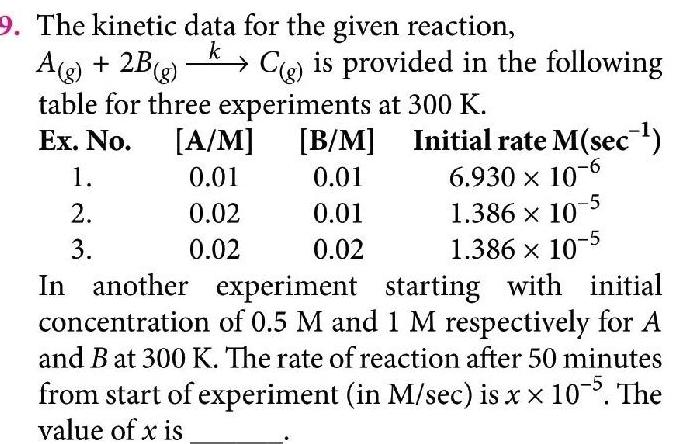Physical Chemistry
Chemical kinetics
9 The kinetic data for the given reaction k A g 2B g table for three experiments at 300 K Ex No A M 0 01 0 02 0 02 1 2 3 C is provided in the following B M Initial rate M sec 0 01 6 930 10 6 0 01 0 02 5 1 386 x 107 1 386 x 10 5 In another experiment starting with initial concentration of 0 5 M and 1 M respectively for A and B at 300 K The rate of reaction after 50 minutes from start of experiment in M sec is x 10 5 The value of x is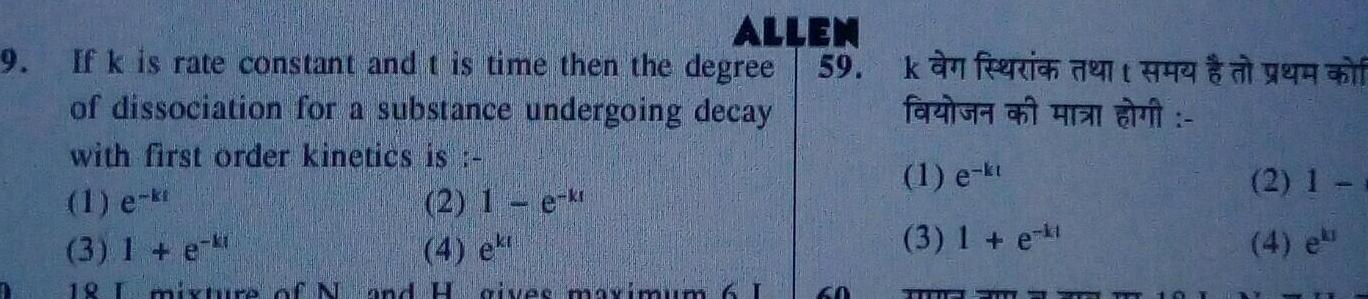Physical Chemistry
Chemical kinetics
ALLEN 9 If k is rate constant and t time then the degree 59 of dissociation for a substance undergoing decay with first order kinetics is 1 e ki 2 1 e kr 4 ekt 3 1 e kt 18 mixure of N and H gives maximum 6 I 60 kan frien farin all it 1 e kt 3 1 e k TE 2 1 4 ek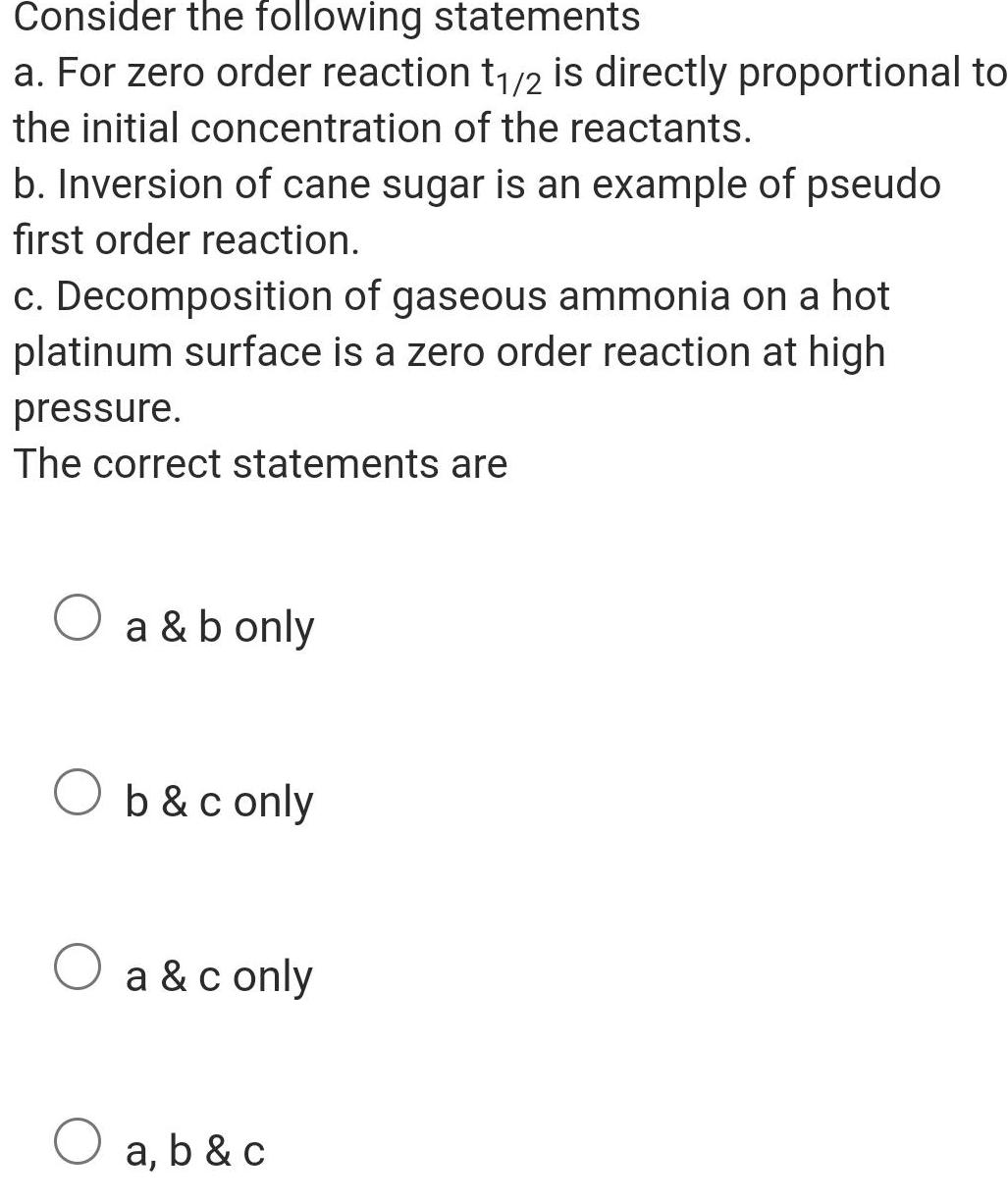Physical Chemistry
Chemical kinetics
Consider the following statements a For zero order reaction t 2 is directly proportional to the initial concentration of the reactants b Inversion of cane sugar is an example of pseudo first order reaction c Decomposition of gaseous ammonia on a hot platinum surface is a zero order reaction at high pressure The correct statements are a b only b c only a c only a b c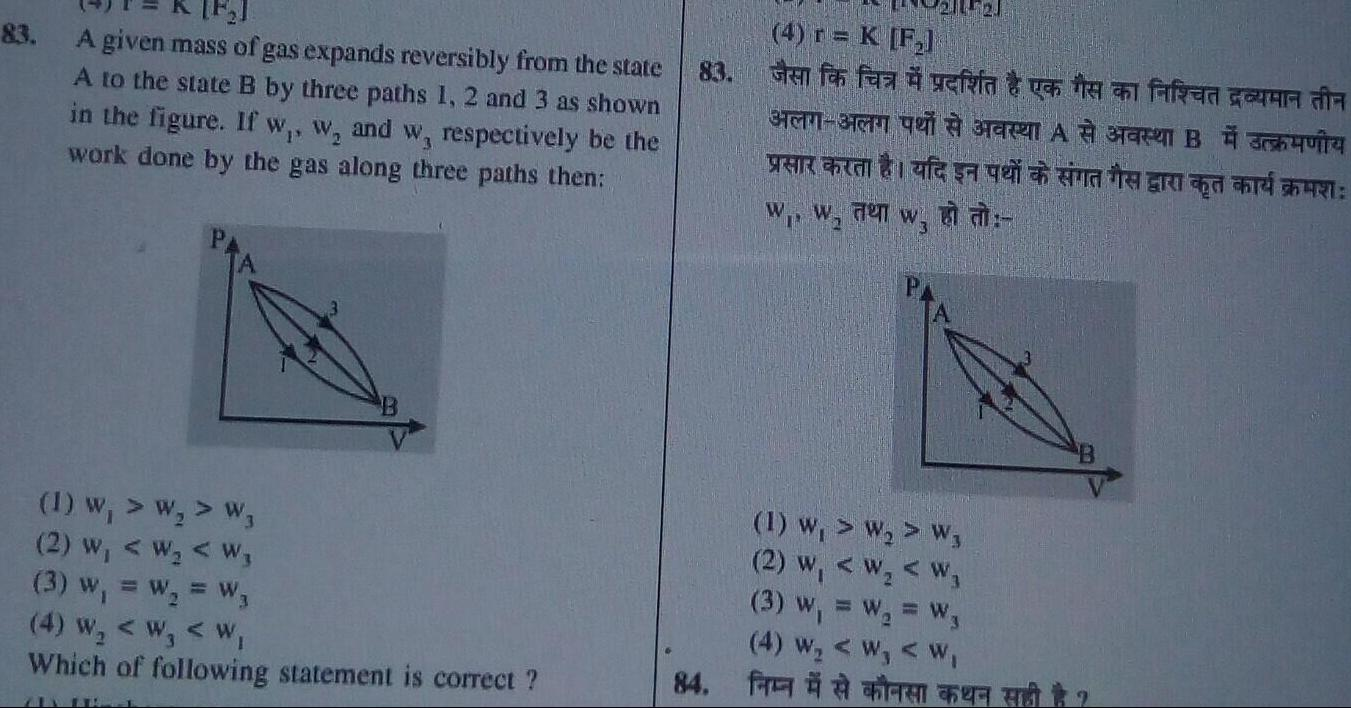Physical Chemistry
Chemical kinetics
83 A given mass of gas expands reversibly from the state A to the state B by three paths 1 2 and 3 as shown in the figure If w w and w respectively be the work done by the gas along three paths then 1 W W W3 2 W W W 3 w W W3 4 W W W Which of following statement is correct 83 84 4 r K F Glen for faa yefia vatten faked un in STRASB CARA W Fen WI 1 W W W3 2 w W W 3 W W W 4 W W W BE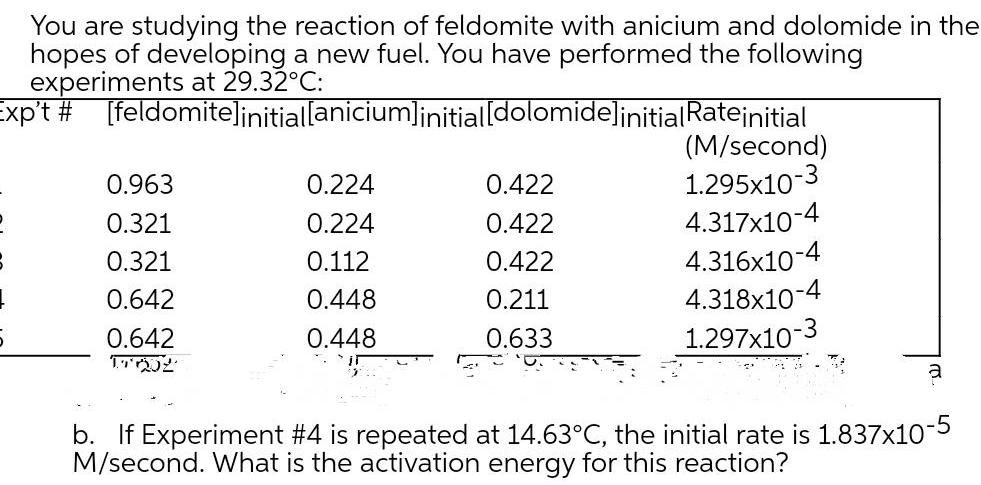Physical Chemistry
Chemical kinetics
You are studying the reaction of feldomite with anicium and dolomide in the hopes of developing a new fuel You have performed the following experiments at 29 32 C Exp t feldomite initial anicium initial dolomide initialRateinitial 0 963 0 321 0 321 0 642 0 642 171552 0 224 0 224 0 112 0 448 0 448 0 422 0 422 0 422 0 211 0 633 M second 1 295x10 3 4 317x10 4 4 316x10 4 4 318x10 4 1 297x10 a b If Experiment 4 is repeated at 14 63 C the initial rate is 1 837x10 5 M second What is the activation energy for this reaction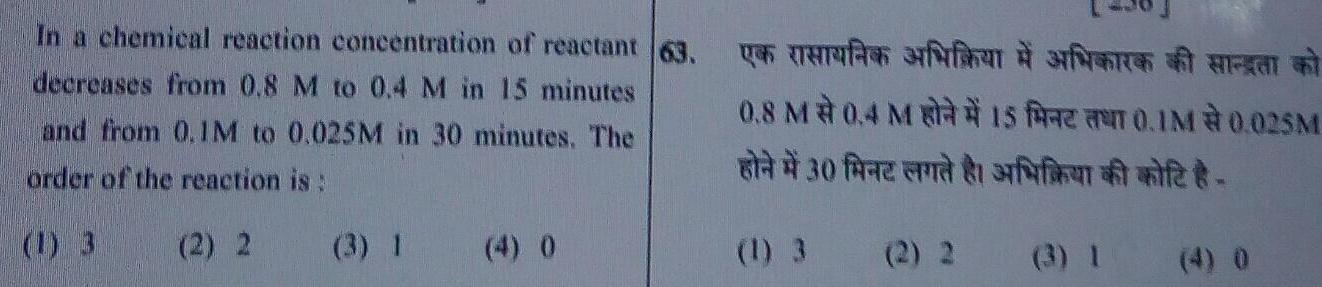Physical Chemistry
Chemical kinetics
In a chemical reaction concentration of reactant 63 decreases from 0 8 M to 0 4 M in 15 minutes and from 0 1M to 0 025M in 30 minutes The order of the reaction is 1 3 2 2 4 0 en 0 8 M0 4 M150 1M 0 025M 30 fe ft 3 1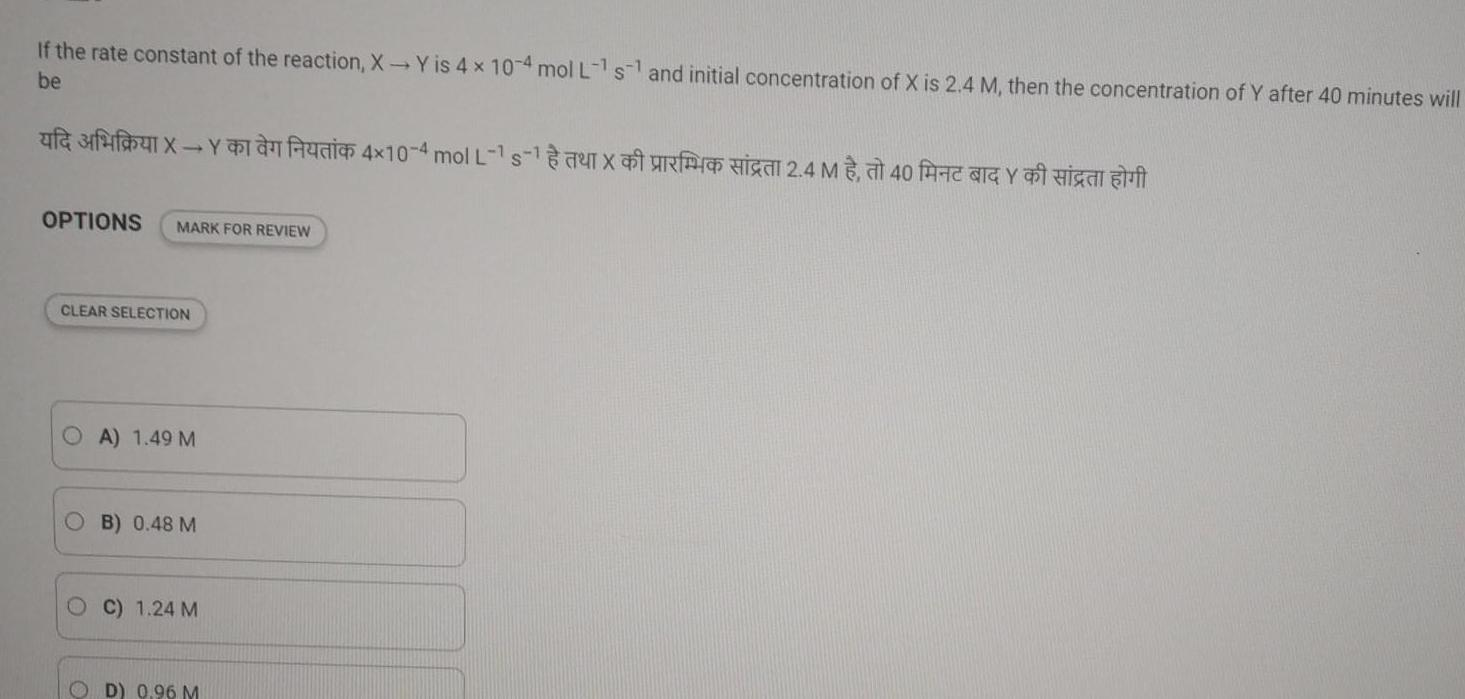Physical Chemistry
Chemical kinetics
If the rate constant of the reaction X Y is 4 x 10 4 mol L 1 s1 and initial concentration of X is 2 4 M then the concentration of Y after 40 minutes will be OPTIONS MARK FOR REVIEW CLEAR SELECTION A 1 49 M OB 0 48 M OC 1 24 M OD 0 96 M 4x10 4 mol L 1s 2 4 M40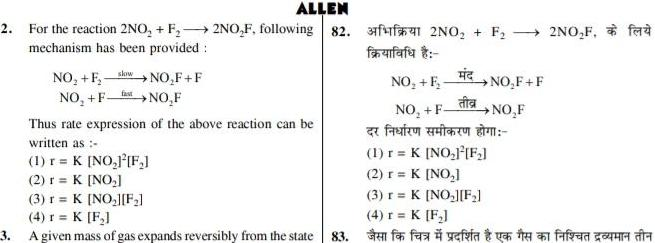Physical Chemistry
Chemical kinetics
ALLEN 2 For the reaction 2NO F 2NO F following 82 mechanism has been provided slow NO F NO F F NO F NO F fast Thus rate expression of the above reaction can be written as 1 r K NO F 2 r K NO 31 2NO F farifafa NO F NO F NO F F NO F 2NO F faz 1 r K NO F 2 r K NO 3 r K NO F 3 r K NO F 4 r K F 4 r K F 3 A given mass of gas expands reversibly from the state 83 ffa eft fafted cour da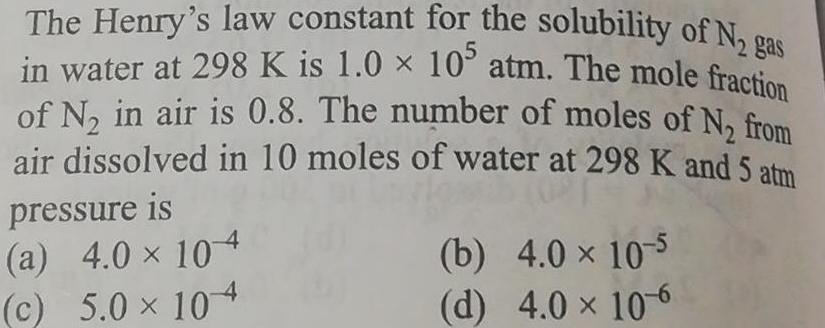Physical Chemistry
Chemical kinetics
The Henry s law constant for the solubility of N gas in water at 298 K is 1 0 x 10 atm The mole fraction of N in air is 0 8 The number of moles of N from air dissolved in 10 moles of water at 298 K and 5 atm pressure is a 4 0 104 c 5 0 104 b 4 0 10 5 d 4 0 10 6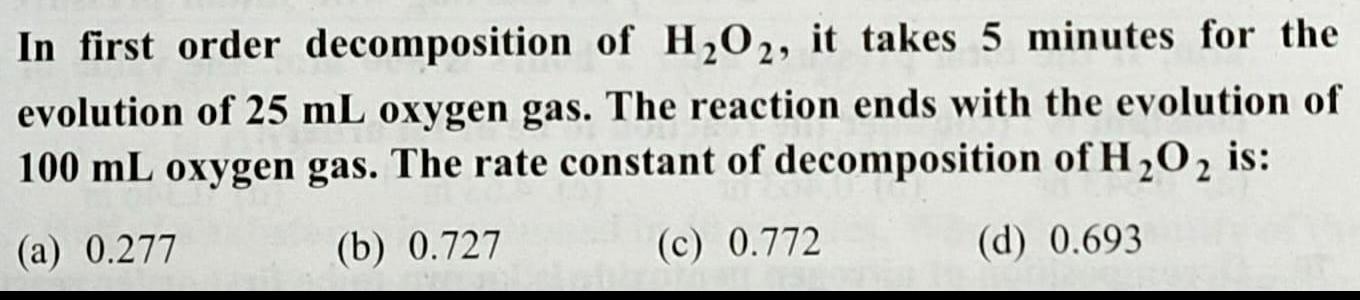Physical Chemistry
Chemical kinetics
In first order decomposition of H O2 it takes 5 minutes for the evolution of 25 mL oxygen gas The reaction ends with the evolution of 100 mL oxygen gas The rate constant of decomposition of H O2 is a 0 277 b 0 727 c 0 772 d 0 693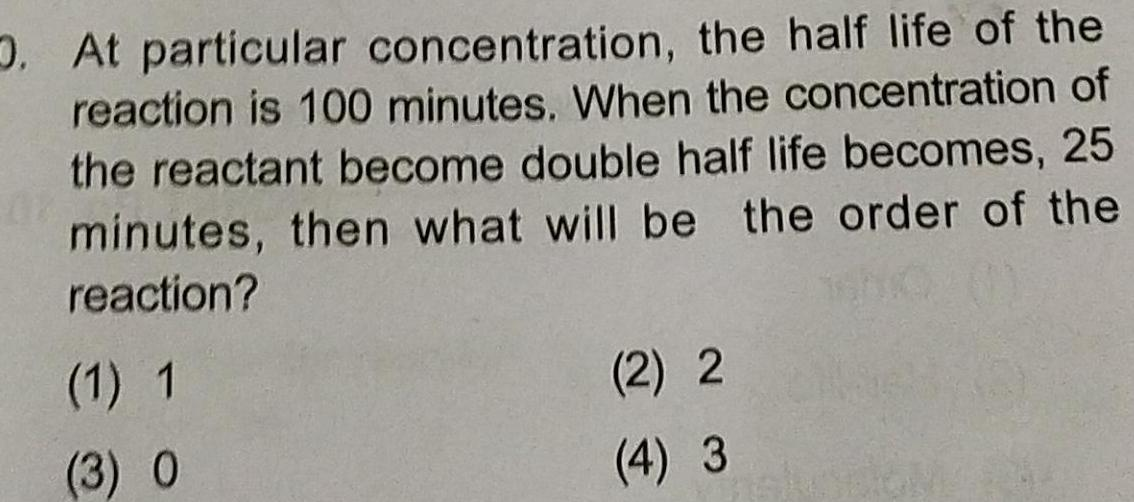Physical Chemistry
Chemical kinetics
O At particular concentration the half life of the reaction is 100 minutes When the concentration of the reactant become double half life becomes 25 minutes then what will be the order of the reaction 1 1 3 O 2 2 4 3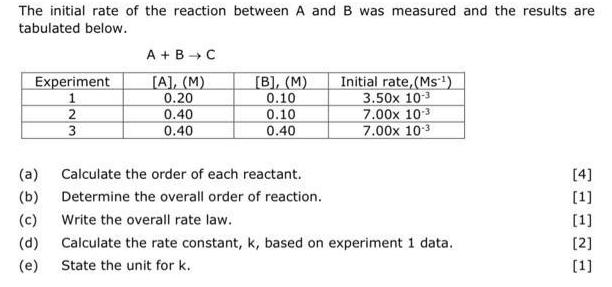Physical Chemistry
Chemical kinetics
The initial rate of the reaction between A and B was measured and the results are tabulated below Experiment 1 2 3 A B C A M 0 20 0 40 0 40 a b c Write the overall rate law d e B M 0 10 0 10 0 40 Calculate the order of each reactant Determine the overall order of reaction Initial rate Ms 3 50x 10 3 7 00x 10 3 7 00x 10 Calculate the rate constant k based on experiment 1 data State the unit for k 4 1 1 2 1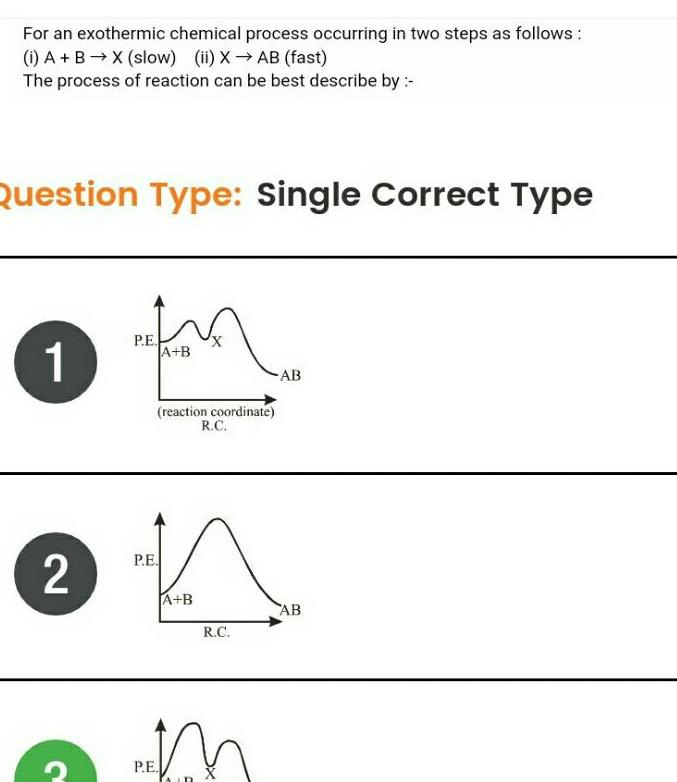Physical Chemistry
Chemical kinetics
For an exothermic chemical process occurring in two steps as follows i A B X slow ii X AB fast The process of reaction can be best describe by Question Type Single Correct Type 1 2 P E A B reaction coordinate R C P E A B P E R C n AB AB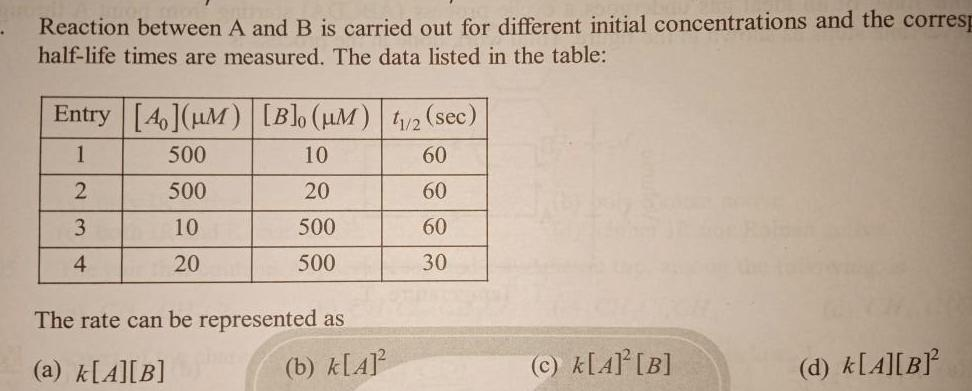Physical Chemistry
Chemical kinetics
Reaction between A and B is carried out for different initial concentrations and the corresp half life times are measured The data listed in the table Entry 40 M Blo UM 1 2 sec 1 500 60 2 60 3 60 4 30 500 10 20 10 20 500 500 The rate can be represented as a k A B b k A c k A B d k A B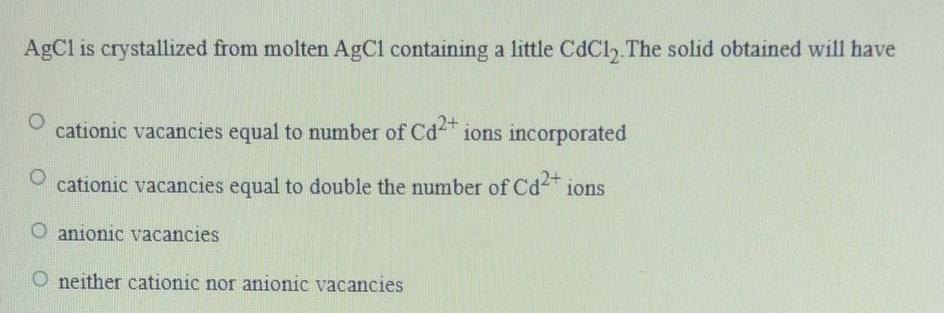Physical Chemistry
Chemical kinetics
AgCl is crystallized from molten AgCl containing a little CdC12 The solid obtained will have cationic vacancies equal to number of Cd ions incorporated cationic vacancies equal to double the number of Cd ions O anionic vacancies neither cationic nor anionic vacancies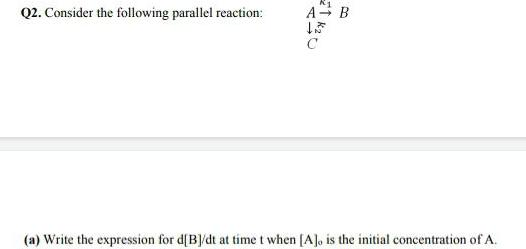Physical Chemistry
Chemical kinetics
Q2 Consider the following parallel reaction A 120 B a Write the expression for d B dt at time t when A is the initial concentration of A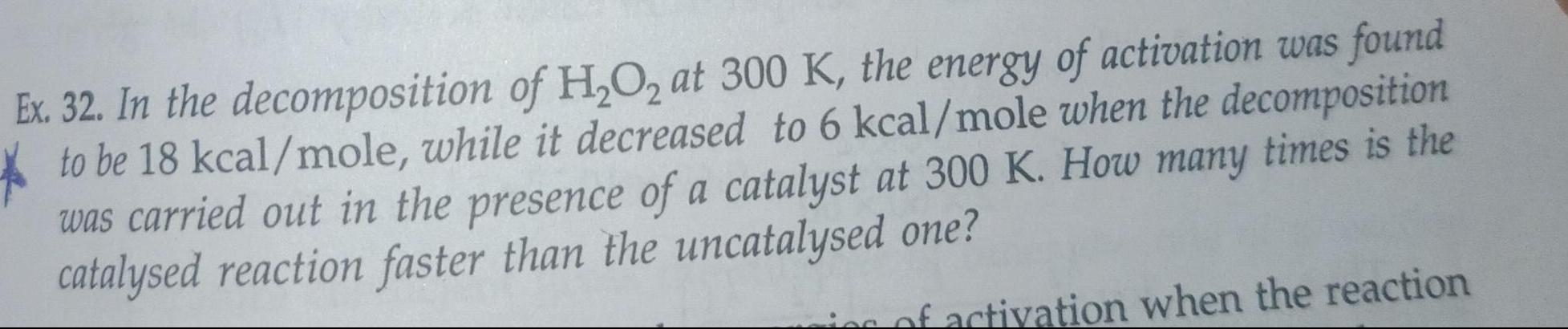Physical Chemistry
Chemical kinetics
Ex 32 In the decomposition of H O2 at 300 K the energy of activation was found to be 18 kcal mole while it decreased to 6 kcal mole when the decomposition was carried out in the presence of a catalyst at 300 K How many times is the catalysed reaction faster than the uncatalysed one ies of activation when the reaction